## NCERT solutions for class 6 mathematics -Exercise Solutions

### Chapter 06-Integers-Exercise Solutions

NCERT Book Page Number-120
Q.1. Write opposites of the following:
(a) Increase in weight
(b) 30 km north
(c) 326 BC
(d) Loss of Rs 700
(e) 100 m above sea level
Answer:
(a) Decrease in weight
(b) 30 km south
(c) 326 A.D.
(d) Gain of Rs 700
(e) 100 m below sea level

Q.2. Represent the following numbers as integers with appropriate signs.
(a) An aeroplane is flying at a height two thousand metre above the ground.
(b) A submarine is moving at a depth, eight hundred metre below the sea level.
(c) A deposit of rupees two hundred.
(d) Withdrawal of rupees seven hundred.
Answer: (a) +2000
(b) −800
(c) +200
(d) −700
Q.3. Represent the following numbers on a number line:
(a) + 5
(b) − 10
(c) + 8
(d) − 1
(e) − 6
Answer:
(a)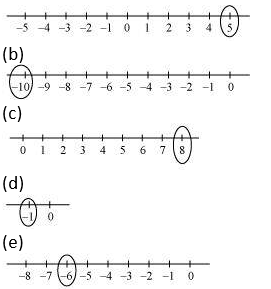Q.4. Adjacent figure is a vertical number line, representing integers. Observe it and locate the following points:
(a) If point D is + 8, then which point is − 8?
(b) Is point G a negative integer or a positive integer?
(c) Write integers for points B and E.
(d) Which point marked on this number line has the least value?
(e) Arrange all the points in decreasing order of value.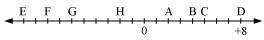Answer:
(a) F
(b) Negative integer (−6)
(c) Point B represents 4 and point E represents −10.
(d) E has the least value as it represents −10.
(e) D > C > B > A > O > H > G > F > E
NCERT Book Page Number-121
Q.5. Following is the list of temperatures of five places in India on a particular day of the year.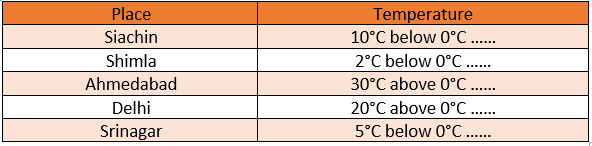(a) Write the temperatures of these places in the form of integers in the blank column.
(b) Following is the number line representing the temperature in degree Celsius.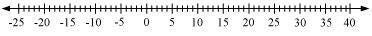Plot the name of the city against its temperature.
(c) Which is the coolest place?
(d) Write the names of the places where temperatures are above 10°C.
Answer:
(a)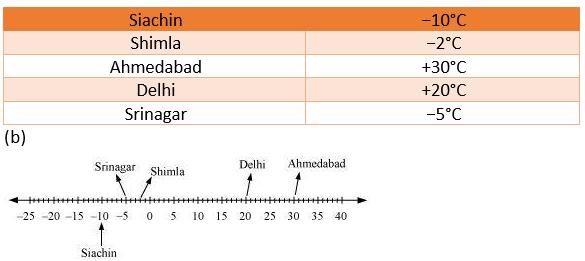(c) Siachin
(d) Delhi, Ahmedabad

Q.6. In each of the following pairs, which number is to the right of the other on the number line?
(a) 2, 9 (b) − 3, − 8 (c) 0, − 1
(d) − 11, 10 (e) − 6, 6 (f) 1, − 100
Answer:
(a) 9 (9 > 2)
(b) −3 (−3 > −8)
(c) 0 (0 > −1)
(d) 10 (10 > −11)
(e) 6 (6 > −6)
(f) 1 (1 > −100)

Q.7. Write all the integers between the given pairs (write them in the increasing order.)
(a) 0 and − 7 (b) − 4 and 4
(c) − 8 and − 15 (d) − 30 and − 23
Answer:
(a) −6, −5, −4, −3, −2, −1
(b) −3, −2, −1, 0, 1, 2, 3
(c) −14, −13, −12, −11, −10, −9
(d) −29, −28, −27, −26, −25, −24

Q.8. (a) Write four negative integers greater than − 20.
(b) Write four negative integers less than − 10.
Answer:
(a) −19, −18, −17, −16
(b) −11, −12, −13, −14

Q.9. For the following statements, write True (T) or False (F). If the statement is false, correct the statement.
(a) − 8 is to the right of − 10 on a number line.
(b) − 100 is to the right of − 50 on a number line.
(c) Smallest negative integer is − 1
(d) − 26 is greater than − 25.
Answer:
(a) True (−8 > −10)
(b) False (−50 > −100)
−100 is to the left of −50 on a number line.
(c) False, as the greatest negative integer is −1.
(d) False, as −26 is smaller than −25.

NCERT Book Page Number-122
Q.10. Draw a number line and answer the following:
(a) Which number will we reach if we move 4 numbers to the right of − 2.
(b) Which number will we reach if we move 5 numbers to the left of 1.
(c) If we are at − 8 on the number line, in which direction should we move to reach − 13?
(d) If we are at − 6 on the number line, in which direction should we move to reach − 1?
Answer:
(a)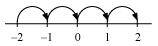We will reach at 2, if we move 4 numbers to the right of −2.
(b)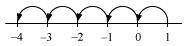We will reach at −4, if we move 5 numbers to the left of 1.
(c)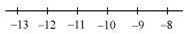Clearly, −13 is to the left of −8. Therefore, we should move towards the left direction.
(d)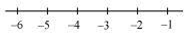Clearly, −1 is to the right of −6. Therefore, we should move towards the right direction.
NCERT Book Page Number-128
Q.1. Using the number line write the integer which is:
(a) 3 more than 5
(b) 5 more than −5
(c) 6 less than 2
(d) 3 less than −2
Answer:
(a)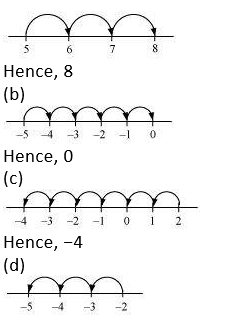Hence, −5
Q.2. Use number line and add the following integers:
(a) 9 + (− 6)
(b) 5 + (− 11)
(c) (− 1) + (− 7)
(d) (− 5) + 10
(e) (− 1) + (− 2) + (− 3)
(f) (− 2) + 8 + (− 4)
Answer:
(a)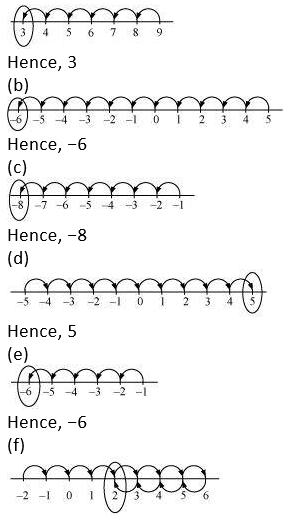Hence, 2
Q.3. Add without using number line:
(a) 11 + (− 7)
(b) (− 13) + (+18)
(c) (− 10) + (+19)
(d) (− 250) + (+ 150)
(e) (− 380) + (− 270)
(f) (− 217) + (− 100)
Answer:
(a) 11 + (−7) = 4
(b) (−13) + (+18) = 5
(c) (−10) + (+19) = 9
(d) (−250) + (+150) = −100
(e) (−380) + (−270) = −650
(f) (−217) + (−100) = −317
NCERT Book Page Number-129
Q.4. (a) 137 and −354 (b) − 52 and 52
(c) − 312, 39 and 192 (d) − 50, − 200 and 300
Answer:
(a) 137 + (−354) = −217
(b) −52 + 52 = 0
(c) −312 + 39 + 192 = −312 + 231 = −81
(d) −50 + (−200) + 300 = −250 + 300 = 50
Q.5. Find the sum:
(a) (− 7) + (− 9) + 4 + 16 (b) (37) + (− 2) + (− 65) + (− 8)
Answer:
(a) (−7) + (−9) + 4 + 16 = −16 + 20 = 4
(b) 37 + (−2) + (−65) + (−8) = 37 + (−75) = −38
NCERT Book Page Number-131
Q.1. Find:
(a) 35 − (20) (b) 72 − (90)
(c) (− 15) − (− 18) (d) (− 20) − (13)
(e) 23 − (− 12) (f) (− 32) − (− 40)
Answer:
(a) 35 − 20 = 15
(b) 72 − 90 = −18
(c) −15 − (−18) = − 15 + 18 = 3
(d) − 20 − 13 = −33
(e) 23 − (−12) = 23 + 12 = 35
(f) (−32) − (−40) = −32 + 40 = 8
Q.2. Fill in the blanks with >, < or = sign.
(a) (− 3) + (− 6) _____ (− 3) − (− 6)
(b) (− 21) − (− 10) _____ (− 31) + (− 11)
(c) 45 − (− 11) _____ 57 + (− 4)
(d) (− 25) − (− 42) _____ (− 42) − (−25)
Answer:
(a) (−3) + (−6) = −9
(−3) − (−6) = − 3 + 6 = 3
−9 < 3
Hence, (− 3) + (− 6) < (− 3) − (− 6) (b) − 21 − (−10) = − 21 + 10 = −11 − 31 + (−11) = −42 −11 > −42
(−21) − (−10) > (−31) + (−11)
(c) 45 − (−11) = 45 + 11 = 56
57 + (−4) = 57 − 4 = 53
56 > 53
45 − (−11) > 57 + (−4)
(d) (−25) − (−42) = − 25 + 42 = 17
− 42 − (−25) = − 42 + 25 = −17
17 > −17
(−25) − (−42) > (−42) − (−25)
Q.3. Fill in the blanks.
(a) (− 8) + _____ = 0
(b) 13 + _____ = 0
(c) 12 + (− 12) = _____
(d) (− 4) + _____ = − 12
(e) _____ − 15 = − 10
Answer:
(a) − 8 + 8 = 0
(b) 13 + (-13) = 0
(c) 12 + (−12) = 0
(d) − 4 + (−8) = −12
(e) 5 − 15 = −10
Q.4. Find:
(a) (− 7) − 8 − (− 25)
(b) (− 13) + 32 − 8 − 1
(c) (− 7) + (− 8) + (− 90)
(d) 50 − (− 40) − (− 2)
Answer:
(a) − 7 − 8 − (−25) = − 7 − 8 + 25 = − 15 + 25 = 10
(b) (−13) + 32 − 8 − 1 = − 13 + 32 − 8 − 1 = 32 − 22 = 10
(c) (−7) + (−8) + (−90) = − 7 − 8 − 90 = −105
(d) 50 − (−40) − (−2) = 50 + 40 + 2 = 92